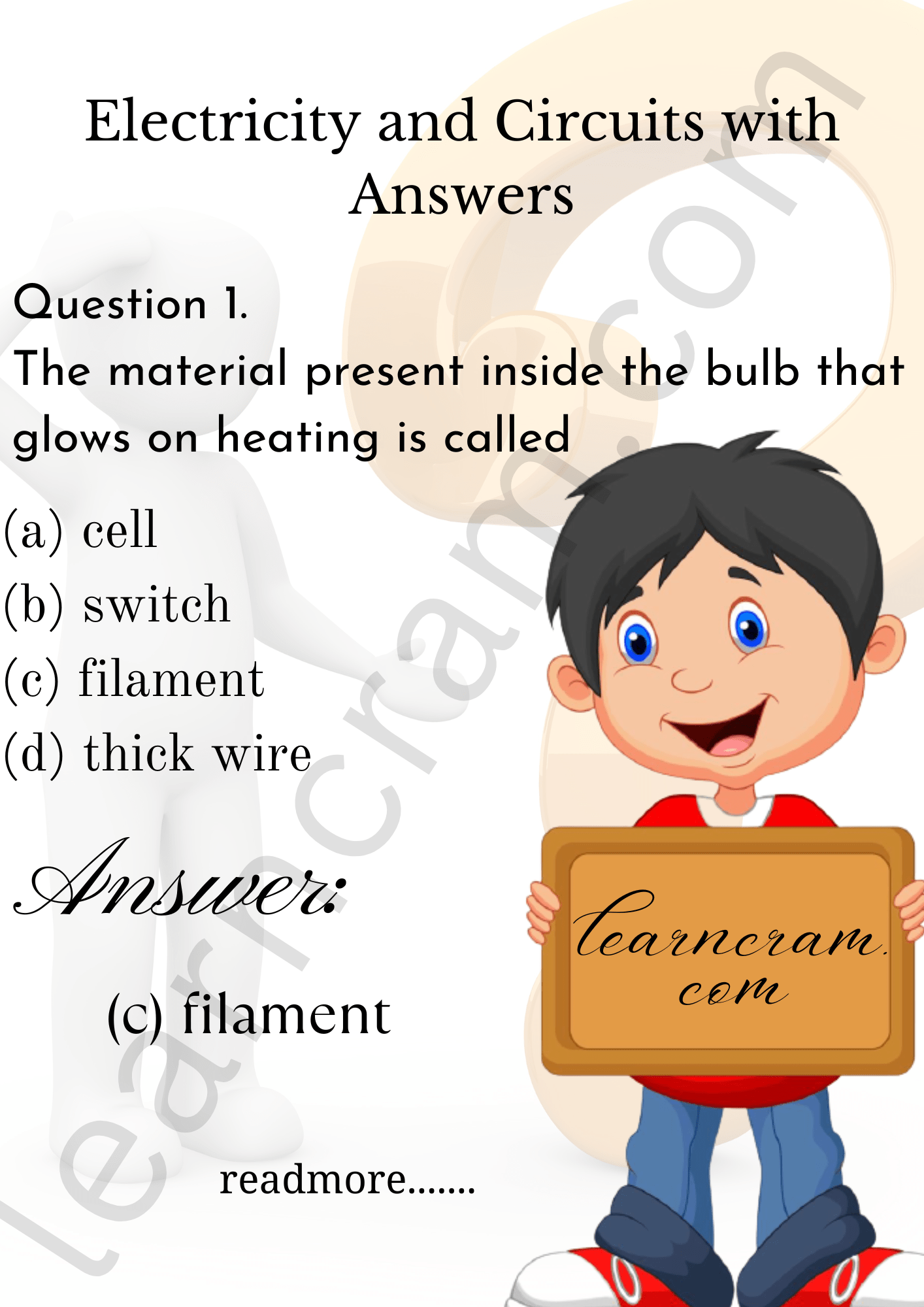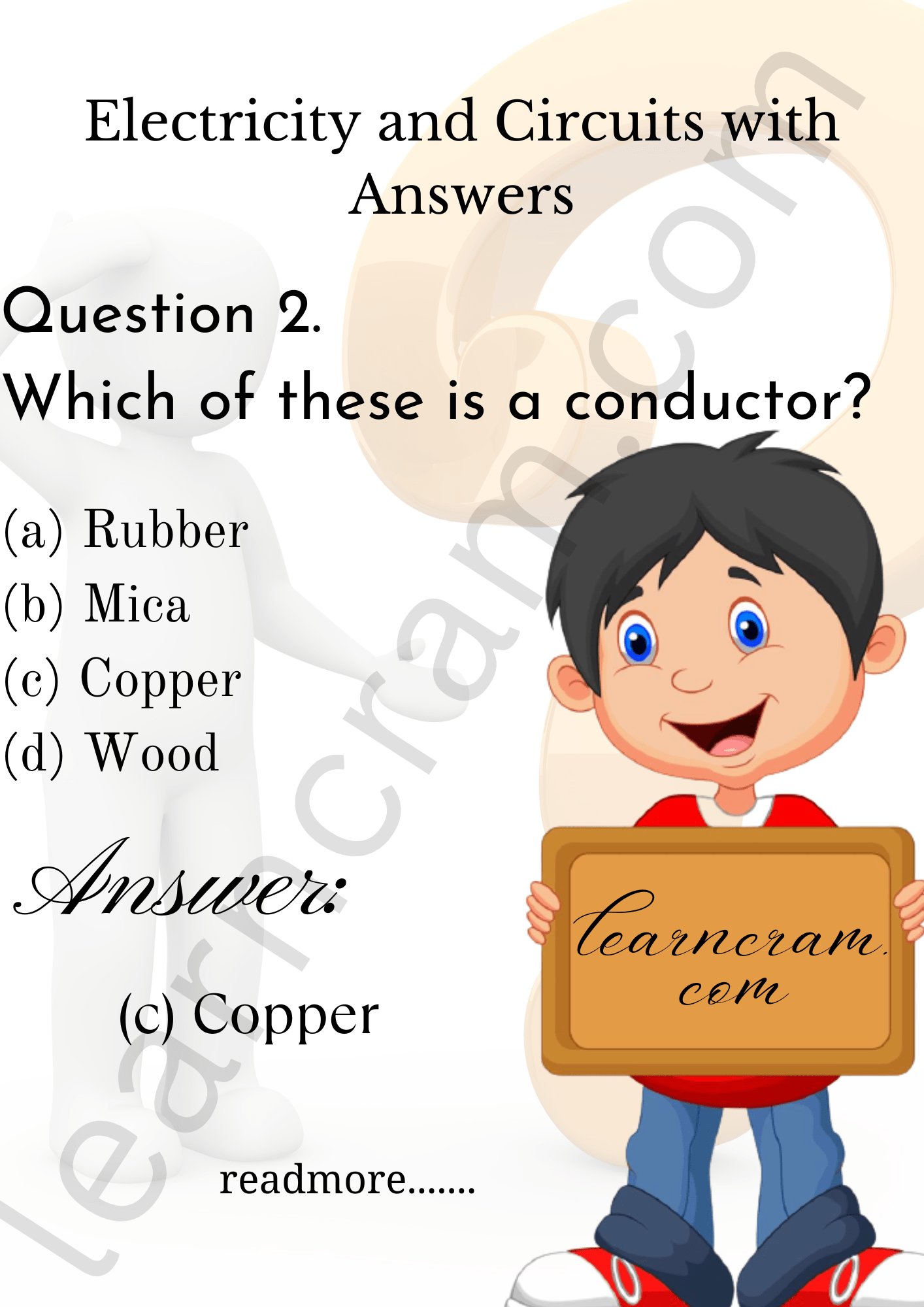# MCQ Questions for Class 6 Science Chapter 12 Electricity and Circuits with Answers

We have compiled the NCERT MCQ Questions for Class 6 Science Chapter 12 Electricity and Circuits with Answers Pdf free download covering the entire syllabus. Practice MCQ Questions for Class 6 Science with Answers on a daily basis and score well in exams. Refer to the Electricity and Circuits Class 6 MCQs Questions with Answers here along with a detailed explanation.

## Electricity and Circuits Class 6 MCQs Questions with Answers

Choose the correct option.

Question 1.
The material present inside the bulb that glows on heating is called
(a) cell
(b) switch
(c) filament
(d) thick wireQuestion 2.
Which of these is a conductor?
(a) Rubber
(b) Mica
(c) Copper
(d) WoodQuestion 3.
Our body is
(a) a good conductor of electricity
(b) sometimes good and sometimes bad conductor
(d) none of these

Answer: (a) a good conductor of electricity

Question 4.
Combination of two or more cells is called
(a) battery
(b) cell
(c) bulb
(d) circuit

Question 5.
Rubber is
(a) a semiconductor
(b) a conductor
(c) an insulator
(d) None of these

Question 6.
A device that breaks the circuit is called:
(a) switch
(b) filament
(c) bulb
(d) battery

Question 7.
A bulb has
(a) two terminals and two filaments
(b) two terminals and a filament
(c) multiple terminals and single filament
(d) single terminal and a filament

Answer: (b) two terminals and a filament

Question 8.
The filament of a bulb is made up of
(a) tungsten
(b) platinum
(c) aluminium
(d) chromium

Question 9.
Which of the following is an insulator?
(a) Bakelite
(b) Aluminium
(c) Tap water
(d) Moist air

Question 10.
A bulb lights up only in
(a) a closed circuit
(b) an open circuit
(c) in both
(d) series connection

Question 11.
Which of the following is a non-conductor or electricity?
(a) Battery
(b) Rubber glove
(c) Metal spoon
(d) Coin

Question 12.
Filament of a torch bulb is
(a) a metal case
(b) metal tip at the centre of the base
(c) two thick wires
(d) a thin wire

Question 13.
A dry cell has
(a) two terminals
(b) one terminal
(c) three terminals
(d) none of these

Question 14.
A bulb whose filament is broken is called
(a) fused
(b) glowing
(c) conductor
(d) none of these

Question 15.
The thin wire that gives off light is called
(a) current
(b) filament
(c) bulb
(d) cell

Question 16.
The direction of flow of an electric current in a conducting wire is from:
(a) negative to positive
(b) positive to negative
(c) sometimes positive and sometimes negative

Question 17.
(a) an insulator
(b) a semiconductor
(c) a conductor
(d) none of these

Question 18.
A filament with low melting point is used in
(a) fuse
(b) electric bulb
(c) electric iron
(d) electric heater

Question 19.
An electrician wears a rubber glove while doing electrical repairs to
(a) save electricity
(b) prevent himself from shock
(c) just make fun
(d) keep away from getting dirty

Answer: (b) prevent himself from shock

Question 20.
Glass is
(a) a conductor
(b) an insulator
(e) both conductor and insulator
(d) semiconductor

Question 21.
Battery is the combination of two or more
(a) cells
(b) bulbs
(c) circuits
(d) none of these

Question 22.
Graphite is a/an
(a) insulator
(b) conductor
(c) both of these
(d) none of these

Question 23.
Plastic is a/an
(a) insulator
(b) conductor
(c) both of these
(d) none of these

Question 24.
The bulb lights up only when
(a) the cell, bulb and the wires make a closed circuit.
(b) there is a gap in the electrical path.
(c) the path starts and ends up at the same terminal of the cell.
(d) the cell, bulb and wires make an open circuit.

Answer: (a) the cell, bulb and the wires make a closed circuit.

Question 25.
Thin wire in the bulb that gives off light is
(a) cell
(b) battery
(c) filament
(d) circuit

Question 26.
The direction of current in a conducting wire is
(a) positive to negative terminal
(b) negative to positive terminal
(c) may vary
(d) none of these

Answer: (a) positive to negative terminal

Question 27.
Which of the following will make a closed circuit?
(a) Eraser
(b) Metal Key
(c) Bulb
(d) Battery

Question 28.
A device that breaks the circuit is called
(a) filament
(b) switch
(c) bulb
(d) battery

Question 29.
The materials which allow electric current to pass through them are
(a) conductors
(b) insulators
(c) semi-conductors
(d) None of these

Question 30.
A circuit may have
(a) only one bulb
(b) only two bulbs
(c) only three bulbs
(d) any number of bulbs

Answer: (d) any number of bulbs

Fill in the Blanks

Question 1.
Gases used in electric bulbs are ………………. gases.

Question 2.
A cell converts ………………. energy into ………………. energy.

Question 3.
The combination of two or more cells in a specific order is called a ……………….

Question 4.
A ………………. is a device which makes or breaks the circuit.

Question 5.
A closed path made for the flow of electric current is called ……………….

Question 6.
Our body is a good ………………. of electricity.

Question 7.
Substances through which an electric current can pass are called ……………….

Question 8.
Substances through which an electric current cannot pass are called ……………….

Question 9.
We use a ………………. to test the conductivity of a material, i.e., whether it is a conductor or an insulator.

Question 10.
Electrical charges that build up on the surface of object being rubbed is called ………………. electricity.

Question 11.
There is a thin wire inside the bulb which emits light is called ………………. of the bulb.

Question 12.
………………. and ………………. are used for covering electrical wires, plug tops, switches and other electrical appliances.

Question 13.
Air is ……………….

Question 14.
An electric current flows from terminal of the electric cell to its ………………. terminal.

Question 15.
A bulb in which the ………………. is broken is called a bulb.

Question 16.
………………… is a useful form of energy.

Question 17.
………………… cell does not contain any liquid chemical.

Question 18.
The metal cap is the ………………… terminal of electric cell.

Question 19.
A single dry cell gives a voltage of ………………… volts.

Question 20.
In ………………… circuit current flows from the positive terminal to its negative terminal.

Question 21.
No ………………… flows in an open circuit.

Question 22.
A ………………… works by opening and closing a gap in an electric circuit.

Question 23.
In a torch two cells are connected to a torch bulb through …………………

Question 24.
Cotton and jute are …………………

Question 25.
Silver and copper are …………………

True or False

Question 1.
Electricity is a form of energy.

Question 2.
In an electric cell, the side with the metal cap is the negative terminal.

Question 3.
The electric cell produces electric current.

Question 4.
Gases filled inside the bulb are nitrogen, argon, krypton, etc.

Question 5.
Electricity can pass through thermocol.

Question 6.
A fused bulb does not glow.

Question 7.
When we rub an object with something, it acquires charge.

Question 8.
Our body is a bad conductor of electricity.

Question 9.
Both electric cells and the bulbs have one terminal each.

Question 10.
In an open circuit, electricity cannot flow.

Question 11.
Electric switches, plugs, sockets, etc., are made of conductors.

Question 12.
Light bulb was invented by Thomas Alva Edison.

Question 13.
In a circuit, electric current flows from positive terminal to negative terminal.

Question 14.
Air is a bad conductor of electricity.

Question 15.
Rubber glove is an example of non-conductor of electricity.

Question 16.
Electricity flows in an open electric circuit.

Question 17.
The black rod in a dry cell is lead rod.

Question 18.
Conductors do not allow the electric current to pass through them.

Question 19.
The circuit in which electrical contact at any point is broken is called on open electric circuit.

Question 20.
An electric cell has four terminals.

Question 21.
Handle of an electrician’s screw driver is made of insulator.

Question 22.
Thin wire in an electric bulb is called filament.

Question 23.
The current flows in closed circuit from +ve to -ve terminal of the cell.

Question 24.
Dry cell converts chemical energy into electrical energy.

Match the following

 Column I Column II 1. Switch (a) Electric current flows in 2. Bulb (b) A device which produces electricity 3. Filament (c) A combination of cells 4. Circuit (d) Does not allow electricity to pass through it 5. Insulator (e) Allows electricity to pass through it 6. Conductor (f) Either breaks or completes a circuit 7. Battery (g) Converts electricity into light 8. Cell (h) Glows when electricity passes in it 9. Fused bulb (i) Is a path of electricity 10. Closed circuit (j) Does not light up